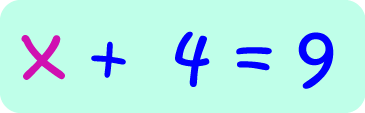# Definition of xWhen we're doing algebra, we often use $x$ to represent something that we don't know the value of.

For example, in the equation $x + 4 = 9$, we don't know the value of $x$, but we can work it out. It should be $5$.

We call $x$ an "unknown", or a "variable".

Variables don't have to be called "$x$". They can be called anything you like. Letters or symbols like "y" and "a" are more common, but $x$ can be anything you like - even "Murgatroyd" or "Engelbert"

### Description

The aim of this dictionary is to provide definitions to common mathematical terms. Students learn a new math skill every week at school, sometimes just before they start a new skill, if they want to look at what a specific term means, this is where this dictionary will become handy and a go-to guide for a student.

### Audience

Year 1 to Year 12 students

### Learning Objectives

Learn common math terms starting with letter X

Author: Subject Coach
You must be logged in as Student to ask a Question.